## Monday, March 12, 2007

### Interest Rate Still Unequal to Marginal Product of Capital (Part 1/2)

1.0 Introduction
I thought I would try to explain again why, in equilibrium, the interest rate is typically unequal to the marginal product of capital. This explanation is based on a model with a different structure than in a previous explanation.

Sometimes you will find, in textbooks and journal articles, claims that, in equilibrium, the interest rate and the marginal product of capital are equal. You may also find economists who claim to be putting forward disagreggated models. They may include production functions in their models with arguments that include the quantities of individual capital goods. Yet those quantities are measured in numeraire units. Such economists are just making a mathematical mistake.

2.0 The Model
Consider a simple economy in a stationary state whose output consists entirely of wood. A worker plants trees at the start of the process of production, which lasts for t years. No labor is required for the trees to grow or to harvest the quantity of wood, q(t), available at the end of t years. Assume that lengthening this process increases the harvest, that is, q'(t) > 0. Also assume that each additional increment of time increases the output less than the previous increment, that is, q''(t) < 0. (This is stronger than needed.) The production function, on a per worker basis, q(t), is graphed in the upper-right quadrant in Figure 1.Figure 1: Optimal Length of Production Dependent on Interest Rate
Assume wages are paid immediately to workers. Competitive firms take the wage, w, and the interest rate, r, as given. Suppose the wage was above the present value of output q(t). The firm could either deposit w in a bank at the interest rate or produce wood. No profit-maximizing firm would do the latter if the wage was this high. But if the wage were below the present value of output, firms would expand production upon seeing an opportunity to make pure economic profit. Thus, competition enforces an equality between the wage and the present value of output.
What is the present value of q(t)? The annual rate of interest is 100 r%. Suppose the interest rate is compounded n times per year. By definition, the per period interest rate is 100 (r/n)%. Table 1 shows the effects of compounding the wage. Since the wage is equal to the present value of output, Equation 1 holds:(1)
Or:(2)
where: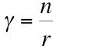(3)
Take the limit as gamma increases without bound, with the process length fixed. One obtains the wage for the case of continuous compounding of interest:(4)
At this point, I have adopted a notation that emphasizes the wage depends on the process length and the interest rate.

Consider a firm producing a stationary state output of q(t). What is the value of the firm's capital at a given point in time? The firm's capital consists of goods in process for producing output from this point until t years in the future. The value of these goods in process is the wages, expended from -t years in the past until the current instant, compounded to that instant:(5)
This integral is easily solved:(6)
Or, using Equation 4: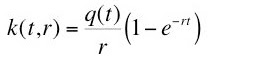(7)
Notice that the wage and the value of capital satisfy an accounting identity: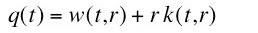(8)

3.0 The Optimal Process Length

The period between planting and harvesting of timber is a choice variable in this model. The firm's managers choose the process length, given the interest rate, r*. From the construction of the so-called factor price frontier, one knows that the optimal technique maximizes the wage. Hence: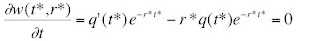(9)
Thus, the optimal length, t*, of the process length is implicitly defined by Equation 10:(10)

One can also find the optimal process length by equating the marginal return to waiting to the marginal cost of borrowing finance capital for the required time increment, dt. The increased output of extending the length of the process used in production, evaluated at the instant in which output originally becomes available, is q(t + dt) - q(t). The extra charge for interest is q(t) r* dt. Equation 11 follows from the usual equilibrium assumption that marginal returns and marginal costs are equal:(11)
Taking the limit as dt approaches zero yields Equation 10. It is not an accident that these two derivations give the same result.

The visualization, in Figure 1, of the choice of the process length, is most easily explained by a transformation of Equation 10. Equation 12 is equivalent to Equation 10: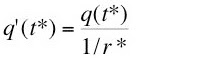(12)
The given interest rate is measured downward from the origin in Figure 1. The
reciprocal of that interest rate is measured leftward from the origin. The southwest quadrant in the figure shows a hyperbola, which relates the interest rate and its reciprocal. The slopes of the parallel lines extending into the northwest quadrant are the ratio of output per worker (measured upward from the origin) and the reciprocal of the interest rate. From Equation 12, one can see that the derivative of the production function is equal to this ratio for the optimal process length. In terms of the geometry, the lower of the two parallel upward-sloping lines is tangent to the production function.